# Tag: Dedekind

Last time we revisited Robin’s theorem saying that 5040 being the largest counterexample to the bound
$\frac{\sigma(n)}{n~log(log(n))} < e^{\gamma} = 1.78107...$ is equivalent to the Riemann hypothesis.

There’s an industry of similar results using other arithmetic functions. Today, we’ll focus on Dedekind’s Psi function
$\Psi(n) = n \prod_{p | n}(1 + \frac{1}{p})$
where $p$ runs over the prime divisors of $n$. It is series A001615 in the online encyclopedia of integer sequences and it starts off with

1, 3, 4, 6, 6, 12, 8, 12, 12, 18, 12, 24, 14, 24, 24, 24, 18, 36, 20, 36, 32, 36, 24, 48, 30, 42, 36, 48, 30, 72, 32, 48, 48, 54, 48, …

and here’s a plot of its first 1000 values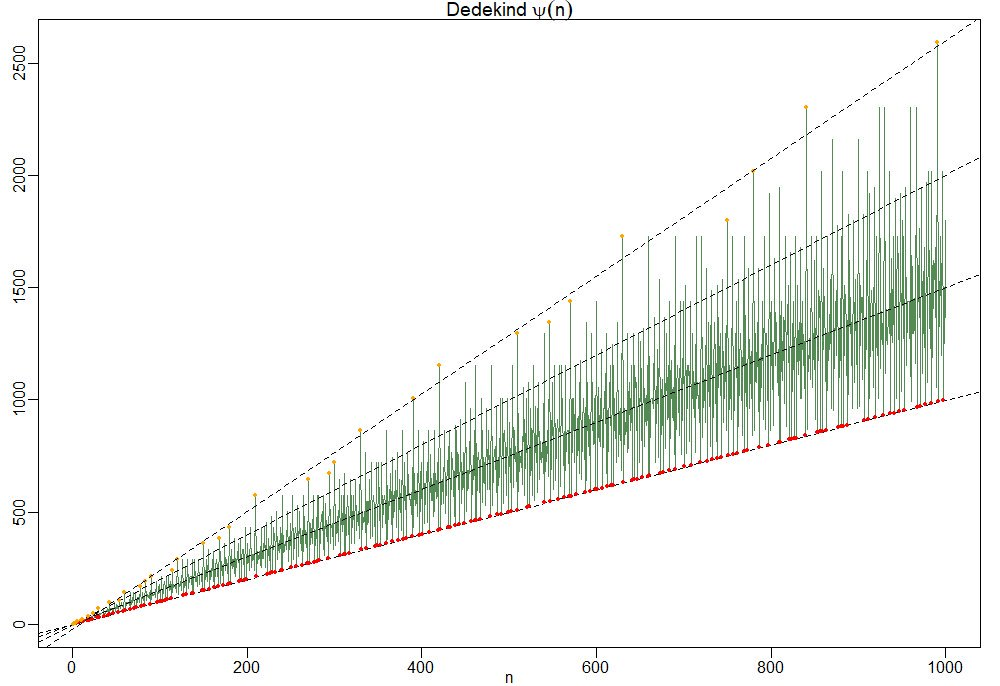To understand this behaviour it is best to focus on the ‘slopes’ $\frac{\Psi(n)}{n}=\prod_{p|n}(1+\frac{1}{p})$.

So, the red dots of minimal ‘slope’ $\approx 1$ correspond to the prime numbers, and the ‘outliers’ have a maximal number of distinct small prime divisors. Look at $210 = 2 \times 3 \times 5 \times 7$ and its multiples $420,630$ and $840$ in the picture.

For this reason the primorial numbers, which are the products of the fist $k$ prime numbers, play a special role. This is series A002110 starting off with

1, 2, 6, 30, 210, 2310, 30030, 510510, 9699690, 223092870,…

In Patrick Solé and Michel Planat Extreme values of the Dedekind $\Psi$ function, it is shown that the primorials play a similar role for Dedekind’s Psi as the superabundant numbers play for the sum-of-divisors function $\sigma(n)$.

That is, if $N_k$ is the $k$-th primorial, then for all $n < N_k$ we have that the 'slope' at $n$ is strictly below that of $N_k$ $\frac{\Psi(n)}{n} < \frac{\Psi(N_k)}{N_k}$ which follows immediately from the fact that any $n < N_k$ can have at most $k-1$ distinct prime factors and $p \mapsto 1 + \frac{1}{p}$ is a strictly decreasing function.

Another easy, but nice, observation is that for all $n$ we have the inequalities
$n^2 > \phi(n) \times \psi(n) > \frac{n^2}{\zeta(2)}$
where $\phi(n)$ is Euler’s totient function
$\phi(n) = n \prod_{p | n}(1 – \frac{1}{p})$
This follows as once from the definitions of $\phi(n)$ and $\Psi(n)$
$\phi(n) \times \Psi(n) = n^2 \prod_{p|n}(1 – \frac{1}{p^2}) < n^2 \prod_{p~\text{prime}} (1 - \frac{1}{p^2}) = \frac{n^2}{\zeta(2)}$ But now it starts getting interesting.

In the proof of his theorem, Guy Robin used a result of his Ph.D. advisor Jean-Louis Nicolasknown as Nicolas’ criterion for the Riemann hypothesis: RH is true if and only if for all $k$ we have the inequality for the $k$-th primorial number $N_k$
$\frac{N_k}{\phi(N_k)~log(log(N_k))} > e^{\gamma}$
From the above lower bound on $\phi(n) \times \Psi(n)$ we have for $n=N_k$ that
$\frac{\Psi(N_k)}{N_k} > \frac{N_k}{\phi(N_k) \zeta(2)}$
and combining this with Nicolas’ criterion we get
$\frac{\Psi(N_k)}{N_k~log(log(N_k))} > \frac{N_k}{\phi(N_k)~log(log(N_k)) \zeta(2)} > \frac{e^{\gamma}}{\zeta(2)} \approx 1.08…$
In fact, Patrick Solé and Michel Planat prove in their paper Extreme values of the Dedekind $\Psi$ function that RH is equivalent to the lower bound
$\frac{\Psi(N_k)}{N_k~log(log(N_k))} > \frac{e^{\gamma}}{\zeta(2)}$
holding for all $k \geq 3$.

Dedekind’s Psi function pops up in lots of interesting mathematics.

In the theory of modular forms, Dedekind himself used it to describe the index of the congruence subgroup $\Gamma_0(n)$ in the full modular group $\Gamma$.

In other words, it gives us the number of tiles needed in the Dedekind tessellation to describe the fundamental domain of the action of $\Gamma_0(n)$ on the upper half-plane by Moebius transformations.When $n=6$ we have $\Psi(6)=12$ and we can view its fundamental domain via these Sage commands:

 G=Gamma0(6) FareySymbol(G).fundamental_domain() 

giving us the 24 back or white tiles (note that these tiles are each fundamental domains of the extended modular group, so we have twice as many of them as for subgroups of the modular group)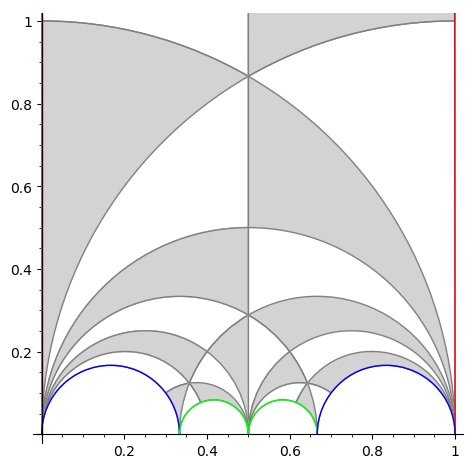But, there are plenty of other, seemingly unrelated, topics where $\Psi(n)$ appears. To name just a few:

• The number of points on the projective line $\mathbb{P}^1(\mathbb{Z}/n\mathbb{Z})$.
• The number of lattices at hyperdistance $n$ in Conway’s big picture.
• The number of admissible maximal commuting sets of operators in the Pauli group for the $n$ qudit.

and there are explicit natural one-to-one correspondences between all these manifestations of $\Psi(n)$, tbc.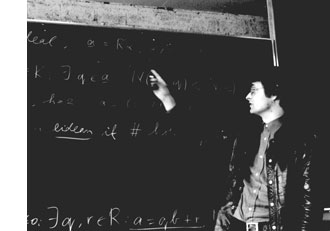Here’s a 5 move game from $\mathbb{C}$, the complex numbers game, annotated by Hendrik Lenstra in Nim multiplication.

$\begin{matrix} & \text{White} & \text{Black} \\ 1. & 3-2i & { 3_{\mathbb{R}} } \\ 2. & 3_{\mathbb{R}} & (22/7)_{\mathbb{Q}} \\ 3. & (-44_{\mathbb{Z}},-14_{\mathbb{Z}})? & { -44_{\mathbb{Z}} } \\ 4. & -44_{\mathbb{Z}} & ( 0_{\mathbb{N}},44_{\mathbb{N}} )! \\ 5. & \text{Resigns} & \\ \end{matrix}$

He writes : “The following 5 comments will make the rules clear.

1 : White selected a complex numbers. Black knows that $\mathbb{C} = \mathbb{R} \times \mathbb{R}$ by $a+bi = (a,b)$, and remembers Kuratowski’s definition of an ordered pair: $~(x,y) = { { x }, { x,y } }$. Thus black must choose an element of ${ { 3_{\mathbb{R}} }, { 3_{\mathbb{R}},-2_{\mathbb{R}} } }$. The index $\mathbb{R}$ here, and later $\mathbb{Q},\mathbb{Z}$ and $\mathbb{N}$, serve to distinguish between real numbers, rational numbers, integers and natural numbers usually denoted by the same symbol. Black’s move leaves White a minimum of choice, but it is not the best one.

2 : White has no choice. The Dedekind definition of $\mathbb{R}$ which the players agreed upon identifies a real number with the set of all strictly larger rational numbers; so Black’s move is legal.

3 : A rational number is an equivalence class of pairs of integers $~(a,b)$ with $b \not= 0$; here $~(a,b)$ represents the rational number $a/b$. The question mark denotes that White’s move is a bad one.

4 : The pair $~(a,b)$ of natural numbers represents the integer $a-b$. Black’s move is the only winning one.

5 : White resigns, since he can choose between ${ 0_{\mathbb{N}} }$ and ${ 0_{\mathbb{N}},44_{\mathbb{N}} }$. In both cases Black will reply by $0_{\mathbb{N}}$, which is the empty set” (and so wins because White has no move left).

These rules make it clear what we mean by the natural numbers $\mathbb{N}$ game, the $\mathbb{Z}$-game and the $\mathbb{Q}$ and $\mathbb{R}$ games. A sum of games is defined as usual (players are allowed to move in exactly one of the component games).

Here’s a 5 term exercise from Lenstra’s paper : Determine the unique winning move in the game $\mathbb{N} + \mathbb{Z} + \mathbb{Q} + \mathbb{R} + \mathbb{C}$

It will take you less than 5 minutes to solve this riddle. Some of the other ‘exercises’ in Lenstra’s paper may take you a lot longer, if not forever…

Exactly 5 years ago I wrote : “As it is probably better to run years behind than to stand eternally still, I’ll try out how much of a blogger I am in 2004.”

5 months ago this became : “from january 1st 2009, I’ll be moving out of here. I will leave the neverendingbooks-site intact for some time to come, so there is no need for you to start archiving it en masse, yet.”

5 minutes before the deadline, this will be my last post….

of 2008

less entropy in 2009!

Last time we tried to generalize the Connes-Consani approach to commutative algebraic geometry over the field with one element $\mathbb{F}_1$ to the noncommutative world by considering covariant functors

$N~:~\mathbf{groups} \rightarrow \mathbf{sets}$

which over $\mathbb{C}$ resp. $\mathbb{Z}$ become visible by a complex (resp. integral) algebra having suitable universal properties.

However, we didn’t specify what we meant by a complex noncommutative variety (resp. an integral noncommutative scheme). In particular, we claimed that the $\mathbb{F}_1$-‘points’ associated to the functor

$D~:~\mathbf{groups} \rightarrow \mathbf{sets} \qquad G \mapsto G_2 \times G_3$ (here $G_n$ denotes all elements of order $n$ of $G$)

were precisely the modular dessins d’enfants of Grothendieck, but didn’t give details. We’ll try to do this now.

For algebras over a field we follow the definition, due to Kontsevich and Soibelman, of so called “noncommutative thin schemes”. Actually, the thinness-condition is implicit in both Soule’s-approach as that of Connes and Consani : we do not consider R-points in general, but only those of rings R which are finite and flat over our basering (or field).

So, what is a noncommutative thin scheme anyway? Well, its a covariant functor (commuting with finite projective limits)

$\mathbb{X}~:~\mathbf{Alg}^{fd}_k \rightarrow \mathbf{sets}$

from finite-dimensional (possibly noncommutative) $k$-algebras to sets. Now, the usual dual-space operator gives an anti-equivalence of categories

$\mathbf{Alg}^{fd}_k \leftrightarrow \mathbf{Coalg}^{fd}_k \qquad A=C^* \leftrightarrow C=A^*$

so a thin scheme can also be viewed as a contra-variant functor (commuting with finite direct limits)

$\mathbb{X}~:~\mathbf{Coalg}^{fd}_k \rightarrow \mathbf{Sets}$

In particular, we are interested to associated to any {tex]k $-algebra$A $its representation functor :$\mathbf{rep}(A)~:~\mathbf{Coalg}^{fd}_k \rightarrow \mathbf{Sets} \qquad C \mapsto Alg_k(A,C^*) $This may look strange at first sight, but$C^* $is a finite dimensional algebra and any$n $-dimensional representation of$A $is an algebra map$A \rightarrow M_n(k) $and we take$C $to be the dual coalgebra of this image. Kontsevich and Soibelman proved that every noncommutative thin scheme$\mathbb{X} $is representable by a$k $-coalgebra. That is, there exists a unique coalgebra$C_{\mathbb{X}} $(which they call the coalgebra of ‘distributions’ of$\mathbb{X} $) such that for every finite dimensional$k $-algebra$B $we have$\mathbb{X}(B) = Coalg_k(B^*,C_{\mathbb{X}}) $In the case of interest to us, that is for the functor$\mathbf{rep}(A) $the coalgebra of distributions is Kostant’s dual coalgebra$A^o $. This is the not the full linear dual of$A $but contains only those linear functionals on$A $which factor through a finite dimensional quotient. So? You’ve exchanged an algebra$A $for some coalgebra$A^o $, but where’s the geometry in all this? Well, let’s look at the commutative case. Suppose$A= \mathbb{C}[X] $is the coordinate ring of a smooth affine variety$X $, then its dual coalgebra looks like$\mathbb{C}[X]^o = \oplus_{x \in X} U(T_x(X)) $the direct sum of all universal (co)algebras of tangent spaces at points$x \in X $. But how do we get the variety out of this? Well, any coalgebra has a coradical (being the sun of all simple subcoalgebras) and in the case just mentioned we have$corad(\mathbb{C}[X]^o) = \oplus_{x \in X} \mathbb{C} e_x $so every point corresponds to a unique simple component of the coradical. In the general case, the coradical of the dual coalgebra$A^o $is the direct sum of all simple finite dimensional representations of$A $. That is, the direct summands of the coalgebra give us a noncommutative variety whose points are the simple representations, and the remainder of the coalgebra of distributions accounts for infinitesimal information on these points (as do the tangent spaces in the commutative case). In fact, it was a surprise to me that one can describe the dual coalgebra quite explicitly, and that$A_{\infty} $-structures make their appearance quite naturally. See this paper if you’re in for the details on this. That settles the problem of what we mean by the noncommutative variety associated to a complex algebra. But what about the integral case? In the above, we used extensively the theory of Kostant-duality which works only for algebras over fields… Well, not quite. In the case of$\mathbb{Z} $(or more general, of Dedekind domains) one can repeat Kostant’s proof word for word provided one takes as the definition of the dual$\mathbb{Z} $-coalgebra of an algebra (which is$\mathbb{Z} $-torsion free)$A^o = { f~:~A \rightarrow \mathbb{Z}~:~A/Ker(f)~\text{is finitely generated and torsion free}~} $(over general rings there may be also variants of this duality, as in Street’s book an Quantum groups). Probably lots of people have come up with this, but the only explicit reference I have is to the first paper I’ve ever written. So, also for algebras over$\mathbb{Z} $we can define a suitable noncommutative integral scheme (the coradical approach accounts only for the maximal ideals rather than all primes, but somehow this is implicit in all approaches as we consider only thin schemes). Fine! So, we can make sense of the noncommutative geometrical objects corresponding to the group-algebras$\mathbb{C} \Gamma $and$\mathbb{Z} \Gamma $where$\Gamma = PSL_2(\mathbb{Z}) $is the modular group (the algebras corresponding to the$G \mapsto G_2 \times G_3 $-functor). But, what might be the points of the noncommutative scheme corresponding to$\mathbb{F}_1 \Gamma $??? Well, let’s continue the path cut out before. “Points” should correspond to finite dimensional “simple representations”. Hence, what are the finite dimensional simple$\mathbb{F}_1 $-representations of$\Gamma $? (Or, for that matter, of any group$G $) Here we come back to Javier’s post on this : a finite dimensional$\mathbb{F}_1 $-vectorspace is a finite set. A$\Gamma $-representation on this set (of n-elements) is a group-morphism$\Gamma \rightarrow GL_n(\mathbb{F}_1) = S_n $hence it gives a permutation representation of$\Gamma $on this set. But then, if finite dimensional$\mathbb{F}_1 $-representations of$\Gamma $are the finite permutation representations, then the simple ones are the transitive permutation representations. That is, the points of the noncommutative scheme corresponding to$\mathbb{F}_1 \Gamma $are the conjugacy classes of subgroups$H \subset \Gamma $such that$\Gamma/H $is finite. But these are exactly the modular dessins d’enfants introduced by Grothendieck as I explained a while back elsewhere (see for example this post and others in the same series). We saw that the icosahedron can be constructed from the alternating group$A_5 $by considering the elements of a conjugacy class of order 5 elements as the vertices and edges between two vertices if their product is still in the conjugacy class. This description is so nice that one would like to have a similar construction for the buckyball. But, the buckyball has 60 vertices, so they surely cannot correspond to the elements of a conjugacy class of$A_5 $. But, perhaps there is a larger group, somewhat naturally containing$A_5 $, having a conjugacy class of 60 elements? This is precisely the statement contained in Galois’ last letter. He showed that 11 is the largest prime p such that the group$L_2(p)=PSL_2(\mathbb{F}_p) $has a (transitive) permutation presentation on p elements. For, p=11 the group$L_2(11) $is of order 660, so it permuting 11 elements means that this set must be of the form$X=L_2(11)/A $with$A \subset L_2(11) $a subgroup of 60 elements… and it turns out that$A \simeq A_5 $… Actually there are TWO conjugacy classes of subgroups isomorphic to$A_5 $in$L_2(11) $and we have already seen one description of these using the biplane geometry (one class is the stabilizer subgroup of a ‘line’, the other the stabilizer subgroup of a point). Here, we will give yet another description of these two classes of$A_5 $in$L_2(11) $, showing among other things that the theory of dessins d’enfant predates Grothendieck by 100 years. In the very same paper containing the first depiction of the Dedekind tessellation, Klein found that there should be a degree 11 cover$\mathbb{P}^1_{\mathbb{C}} \rightarrow \mathbb{P}^1_{\mathbb{C}} $with monodromy group$L_2(11) $, ramified only in the three points${ 0,1,\infty } $such that there is just one point lying over$\infty $, seven over 1 of which four points where two sheets come together and finally 5 points lying over 0 of which three where three sheets come together. In 1879 he wanted to determine this cover explicitly in the paper “Ueber die Transformationen elfter Ordnung der elliptischen Funktionen” (Math. Annalen) by describing all Riemann surfaces with this ramification data and pick out those with the correct monodromy group.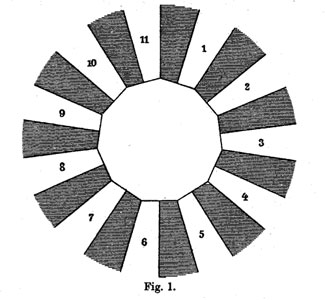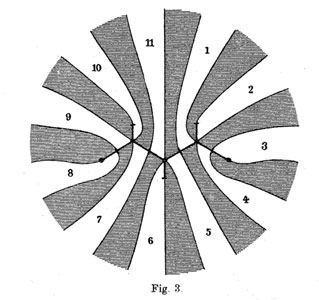He manages to do so by associating to all these covers their ‘dessins d’enfants’ (which he calls Linienzuges), that is the pre-image of the interval [0,1] in which he marks the preimages of 0 by a bullet and those of 1 by a +, such as in the innermost darker graph on the right above. He even has these two wonderful pictures explaining how the dessin determines how the 11 sheets fit together. (More examples of dessins and the correspondences of sheets were drawn in the 1878 paper.)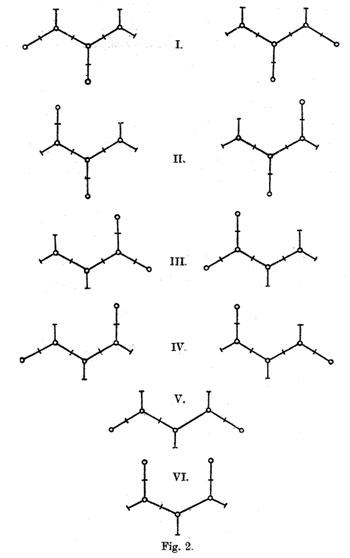The ramification data translates to the following statements about the Linienzuge : (a) it must be a tree ($\infty $has one preimage), (b) there are exactly 11 (half)edges (the degree of the cover), (c) there are 7 +-vertices and 5 o-vertices (preimages of 0 and 1) and (d) there are 3 trivalent o-vertices and 4 bivalent +-vertices (the sheet-information). Klein finds that there are exactly 10 such dessins and lists them in his Fig. 2 (left). Then, he claims that one the two dessins of type I give the correct monodromy group. Recall that the monodromy group is found by giving each of the half-edges a number from 1 to 11 and looking at the permutation$\tau $of order two pairing the half-edges adjacent to a +-vertex and the order three permutation$\sigma $listing the half-edges by cycling counter-clockwise around a o-vertex. The monodromy group is the group generated by these two elements. Fpr example, if we label the type V-dessin by the numbers of the white regions bordering the half-edges (as in the picture Fig. 3 on the right above) we get$\sigma = (7,10,9)(5,11,6)(1,4,2) $and$\tau=(8,9)(7,11)(1,5)(3,4) $. Nowadays, it is a matter of a few seconds to determine the monodromy group using GAP and we verify that this group is$A_{11} $. Of course, Klein didn’t have GAP at his disposal, so he had to rule out all these cases by hand. gap> g:=Group((7,10,9)(5,11,6)(1,4,2),(8,9)(7,11)(1,5)(3,4)); Group([ (1,4,2)(5,11,6)(7,10,9), (1,5)(3,4)(7,11)(8,9) ]) gap> Size(g); 19958400 gap> IsSimpleGroup(g); true Klein used the fact that$L_2(11) $only has elements of orders 1,2,3,5,6 and 11. So, in each of the remaining cases he had to find an element of a different order. For example, in type V he verified that the element$\tau.(\sigma.\tau)^3 $is equal to the permutation (1,8)(2,10,11,9,6,4,5)(3,7) and consequently is of order 14. Perhaps Klein knew this but GAP tells us that the monodromy group of all the remaining 8 cases is isomorphic to the alternating group$A_{11} $and in the two type I cases is indeed$L_2(11) $. Anyway, the two dessins of type I correspond to the two conjugacy classes of subgroups$A_5 $in the group$L_2(11) $. But, back to the buckyball! The upshot of all this is that we have the group$L_2(11) $containing two classes of subgroups isomorphic to$A_5 $and the larger group$L_2(11) $does indeed have two conjugacy classes of order 11 elements containing exactly 60 elements (compare this to the two conjugacy classes of order 5 elements in$A_5 $in the icosahedral construction). Can we construct the buckyball out of such a conjugacy class? To start, we can identify the 12 pentagons of the buckyball from a conjugacy class C of order 11 elements. If$x \in C $, then so do$x^3,x^4,x^5 $and$x^9 $, whereas the powers${ x^2,x^6,x^7,x^8,x^{10} } $belong to the other conjugacy class. Hence, we can divide our 60 elements in 12 subsets of 5 elements and taking an element x in each of these, the vertices of a pentagon correspond (in order) to$~(x,x^3,x^9,x^5,x^4) $. Group-theoretically this follows from the fact that the factorgroup of the normalizer of x modulo the centralizer of x is cyclic of order 5 and this group acts naturally on the conjugacy class of x with orbits of size 5. Finding out how these pentagons fit together using hexagons is a lot subtler… and in The graph of the truncated icosahedron and the last letter of Galois Bertram Kostant shows how to do this.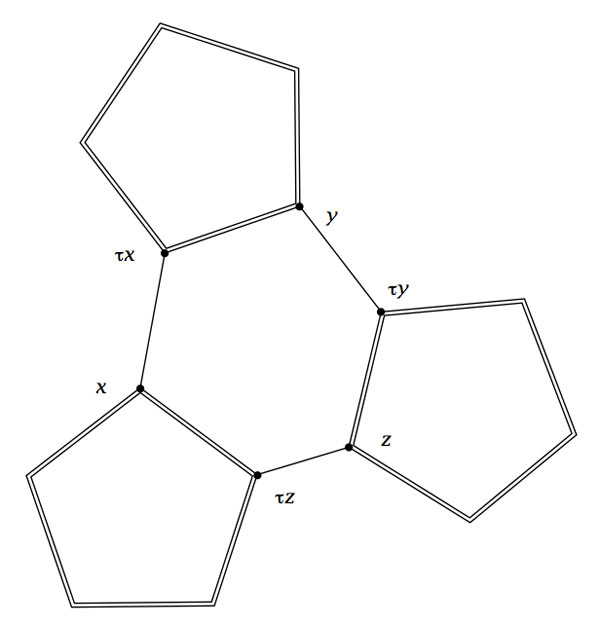Fix a subgroup isomorphic to$A_5 $and let D be the set of all its order 2 elements (recall that they form a full conjugacy class in this$A_5 $and that there are precisely 15 of them). Now, the startling observation made by Kostant is that for our order 11 element$x $in C there is a unique element$a \in D $such that the commutator$~b=[x,a]=x^{-1}a^{-1}xa $belongs again to D. The unique hexagonal side having vertex x connects it to the element$b.x $which belongs again to C as$b.x=(ax)^{-1}.x.(ax) $. Concluding, if C is a conjugacy class of order 11 elements in$L_2(11) $, then its 60 elements can be viewed as corresponding to the vertices of the buckyball. Any element$x \in C $is connected by two pentagonal sides to the elements$x^{3} $and$x^4 $and one hexagonal side connecting it to$\tau x = b.x $. Exactly one year ago this blog was briefly renamed MoonshineMath. The concept being that it would focus on the mathematics surrounding the monster group & moonshine. Well, I got as far as the Mathieu groups… After a couple of months, I changed the name back to neverendingbooks because I needed the freedom to post on any topic I wanted. I know some people preferred the name MoonshineMath, but so be it, anyone’s free to borrow that name for his/her own blog. Today it’s bloomsday again, and, as I’m a cyclical guy, I have another idea for a conceptual blog : the bistromath chronicles (or something along this line). Here’s the relevant section from the Hitchhikers guide Bistromathics itself is simply a revolutionary new way of understanding the behavior of numbers. … Numbers written on restaurant checks within the confines of restaurants do not follow the same mathematical laws as numbers written on any other pieces of paper in any other parts of the Universe. This single statement took the scientific world by storm. It completely revolutionized it.So many mathematical conferences got hold in such good restaurants that many of the finest minds of a generation died of obesity and heart failure and the science of math was put back by years. Right, so what’s the idea? Well, on numerous occasions Ive stated that any math-blog can only survive as a group-blog. I did approach a lot of people directly, but, as you have noticed, without too much success… Most of them couldnt see themselves contributing to a blog for one of these reasons : it costs too much energy and/or it’s way too inefficient. They say : career-wise there are far cleverer ways to spend my energy than to write a blog. And… there’s no way I can argue against this. Whence plan B : set up a group-blog for a fixed amount of time (say one year), expect contributors to write one or two series of about 4 posts on their chosen topic, re-edit the better series afterwards and turn them into a book. But, in order to make a coherent book proposal out of blog-post-series, they’d better center around a common theme, whence the BistroMath ploy. Imagine that some of these forgotten “restaurant-check-notes” are discovered, decoded and explained. Apart from the mathematics, one is free to invent new recepies or add descriptions of restaurants with some mathematical history, etc. etc. One possible scenario (but I’m sure you will have much better ideas) : part of the knotation is found on a restaurant-check of some Italian restaurant. This allow to explain Conway’s theory of rational tangles, give the perfect way to cook spaghetti to experiment with tangles and tell the history of Manin’s Italian restaurant in Bonn where (it is rumoured) the 1998 Fields medals were decided… But then, there is no limit to your imagination as long as it somewhat fits within the framework. For example, I’d love to read the transcripts of a chat-session in SecondLife between Dedekind and Conway on the construction of real numbers… I hope you get the drift. I’m not going to rename neverendingbooks again, but am willing to set up the BistroMath blog provided • Five to ten people are interested to participate • At least one book-editor shows an interest update : (16/06) contacted by first publisher You can leave a comment or, if you prefer, contact me via email (if you’re human you will have no problem getting my address…). Clearly, people already blogging are invited and are allowed to cross-post (in fact, that’s what I will do if it ever gets so far). Finally, if you are not willing to contribute blog-posts but like the idea and are willing to contribute to it in any other way, we are still auditioning for chanting monks The small group of monks who had taken up hanging around the major research institutes singing strange chants to the effect that the Universe was only a figment of its own imagination were eventually given a street theater grant and went away. And, if you do not like this idea, there will be another bloomsday-idea next year…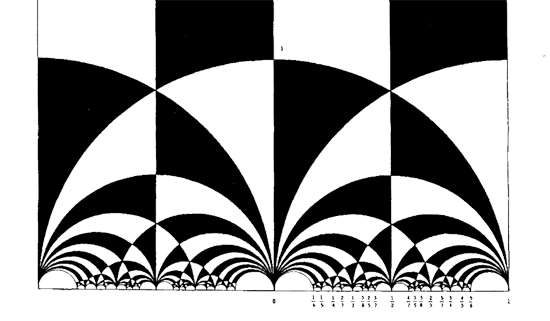The black&white psychedelic picture on the left of a tessellation of the hyperbolic upper-halfplane, was called the Dedekind tessellation in this post, following the reference given by John Stillwell in his excellent paper Modular Miracles, The American Mathematical Monthly, 108 (2001) 70-76. But is this correct terminology? Nobody else uses it apparently. So, let’s try to track down the earliest depiction of this tessellation in the literature…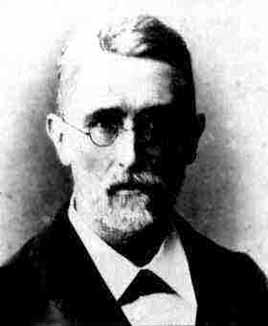Stillwell refers to Richard Dedekind‘s 1877 paper “Schreiben an Herrn Borchard uber die Theorie der elliptische Modulfunktionen”, which appeared beginning of september 1877 in Crelle’s journal (Journal fur die reine und angewandte Mathematik, Bd. 83, 265-292). There are a few odd things about this paper. To start, it really is the transcript of a (lengthy) letter to Herrn Borchardt (at first, I misread the recipient as Herrn Borcherds which would be really weird…), written on June 12th 1877, just 2 and a half months before it appeared… Even today in the age of camera-ready-copy it would probably take longer. There isn’t a single figure in the paper, but, it is almost impossible to follow Dedekind’s arguments without having a mental image of the tessellation. He gives a fundamental domain for the action of the modular group$\Gamma = PSL_2(\mathbb{Z}) $on the hyperbolic upper-half plane (a fact already known to Gauss) and goes on in section 3 to give a one-to-one mapping between this domain and the complex plane using what he calls the ‘valenz’ function$v $(which is our modular function$j $, making an appearance in moonshine, and responsible for the black&white tessellation, the two colours corresponding to pre-images of the upper or lower half-planes). Then there is this remarkable opening sentence. Sie haben mich aufgefordert, eine etwas ausfuhrlichere Darstellung der Untersuchungen auszuarbeiten, von welchen ich, durch das Erscheinen der Abhandlung von Fuchs veranlasst, mir neulich erlaubt habe Ihnen eine kurze Ubersicht mitzuteilen; indem ich Ihrer Einladung hiermit Folge leiste, beschranke ich mich im wesentlichen auf den Teil dieser Untersuchungen, welcher mit der eben genannten Abhandlung zusammenhangt, und ich bitte Sie auch, die Ubergehung einiger Nebenpunkte entschuldigen zu wollen, da es mir im Augenblick an Zeit fehlt, alle Einzelheiten auszufuhren. Well, just try to get a paper (let alone a letter) accepted by Crelle’s Journal with an opening line like : “I’ll restrict to just a few of the things I know, and even then, I cannot be bothered to fill in details as I don’t have the time to do so right now!” But somehow, Dedekind got away with it. So, who was this guy Borchardt? How could this paper be published so swiftly? And, what might explain this extreme ‘je m’en fous’-opening ?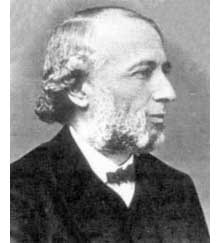Carl Borchardt was a Berlin mathematician whose main claim to fame seems to be that he succeeded Crelle in 1856 as main editor of the ‘Journal fur reine und…’ until 1880 (so in 1877 he was still in charge, explaining the swift publication). It seems that during this time the ‘Journal’ was often referred to as “Borchardt’s Journal” or in France as “Journal de M Borchardt”. After Borchardt’s death, the Journal für die Reine und Angewandte Mathematik again became known as Crelle’s Journal. As to the opening sentence, I have a toy-theory of what was going on. In 1877 a bitter dispute was raging between Kronecker (an editor for the Journal and an important one as he was the one succeeding Borchardt when he died in 1880) and Cantor. Cantor had published most of his papers at Crelle and submitted his latest find : there is a one-to-one correspondence between points in the unit interval [0,1] and points of d-dimensional space! Kronecker did everything in his power to stop that paper to the extend that Cantor wanted to retract it and submit it elsewhere. Dedekind supported Cantor and convinced him not to retract the paper and used his influence to have the paper published in Crelle in 1878. Cantor greatly resented Kronecker’s opposition to his work and never submitted any further papers to Crelle’s Journal. Clearly, Borchardt was involved in the dispute and it is plausible that he ‘invited’ Dedekind to submit a paper on his old results in the process. As a further peace offering, Dedekind included a few ‘nice’ words for Kronecker Bei meiner Versuchen, tiefer in diese mir unentbehrliche Theorie einzudringen und mir einen einfachen Weg zu den ausgezeichnet schonen Resultaten von Kronecker zu bahnen, die leider noch immer so schwer zuganglich sind, enkannte ich sogleich… Probably, Dedekind was referring to Kronecker’s relation between class groups of quadratic imaginary fields and the j-function, see the miracle of 163. As an added bonus, Dedekind was elected to the Berlin academy in 1880… Anyhow, no visible sign of ‘Dedekind’s’ tessellation in the 1877 Dedekind paper, so, we have to look further. I’m fairly certain to have found the earliest depiction of the black&white tessellation (if you have better info, please drop a line). Here it is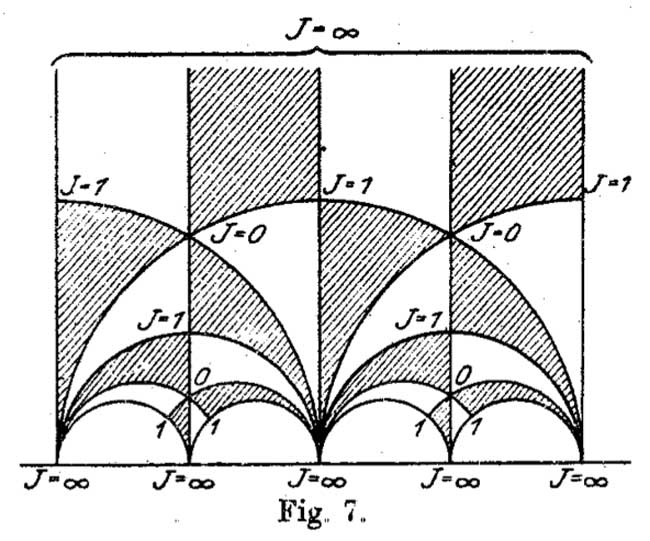It is figure 7 in Felix Klein‘s paper “Uber die Transformation der elliptischen Funktionen und die Auflosung der Gleichungen funften Grades” which appeared in may 1878 in the Mathematische Annalen (Bd. 14 1878/79). He even adds the j-values which make it clear why black triangles should be oriented counter-clockwise and white triangles clockwise. If Klein would still be around today, I’m certain he’d be a metapost-guru. So, perhaps the tessellation should be called Klein’s tessellation?? Well, not quite. Here’s what Klein writes wrt. figure 7 Diese Figur nun – welche die eigentliche Grundlage fur das Nachfolgende abgibt – ist eben diejenige, von der Dedekind bei seiner Darstellung ausgeht. Er kommt zu ihr durch rein arithmetische Betrachtung. Case closed : Klein clearly acknowledges that Dedekind did have this picture in mind when writing his 1877 paper! But then, there are a few odd things about Klein’s paper too, and, I do have a toy-theory about this as well… (tbc) John Conway once wrote : There are almost as many different constructions of$M_{24} $as there have been mathematicians interested in that most remarkable of all finite groups. In the inguanodon post Ive added yet another construction of the Mathieu groups$M_{12} $and$M_{24} $starting from (half of) the Farey sequences and the associated cuboid tree diagram obtained by demanding that all edges are odd. In this way the Mathieu groups turned out to be part of a (conjecturally) infinite sequence of simple groups, starting as follows :$L_2(7),M_{12},A_{16},M_{24},A_{28},A_{40},A_{48},A_{60},A_{68},A_{88},A_{96},A_{120},A_{132},A_{148},A_{164},A_{196},\ldots $It is quite easy to show that none of the other sporadics will appear in this sequence via their known permutation representations. Still, several of the sporadic simple groups are generated by an element of order two and one of order three, so they are determined by a finite dimensional permutation representation of the modular group$PSL_2(\mathbb{Z}) $and hence are hiding in a special polygonal region of the Dedekind’s tessellationLet us try to figure out where the sporadic with the next simplest permutation representation is hiding : the second Janko group$J_2 $, via its 100-dimensional permutation representation. The Atlas tells us that the order two and three generators act as e:= (1,84)(2,20)(3,48)(4,56)(5,82)(6,67)(7,55)(8,41)(9,35)(10,40)(11,78)(12, 100)(13,49)(14,37)(15,94)(16,76)(17,19)(18,44)(21,34)(22,85)(23,92)(24, 57)(25,75)(26,28)(27,64)(29,90)(30,97)(31,38)(32,68)(33,69)(36,53)(39,61) (42,73)(43,91)(45,86)(46,81)(47,89)(50,93)(51,96)(52,72)(54,74)(58,99) (59,95)(60,63)(62,83)(65,70)(66,88)(71,87)(77,98)(79,80); v:= (1,80,22)(2,9,11)(3,53,87)(4,23,78)(5,51,18)(6,37,24)(8,27,60)(10,62,47) (12,65,31)(13,64,19)(14,61,52)(15,98,25)(16,73,32)(17,39,33)(20,97,58) (21,96,67)(26,93,99)(28,57,35)(29,71,55)(30,69,45)(34,86,82)(38,59,94) (40,43,91)(42,68,44)(46,85,89)(48,76,90)(49,92,77)(50,66,88)(54,95,56) (63,74,72)(70,81,75)(79,100,83);  But as the kfarey.sage package written by Chris Kurth calculates the Farey symbol using the L-R generators, we use GAP to find those L = e*v^-1 and R=e*v^-2 so L=(1,84,22,46,70,12,79)(2,58,93,88,50,26,35)(3,90,55,7,71,53,36)(4,95,38,65,75,98,92)(5,86,69,39,14,6,96)(8,41,60,72,61,17, 64)(9,57,37,52,74,56,78)(10,91,40,47,85,80,83)(11,23,49,19,33,30,20)(13,77,15,59,54,63,27)(16,48,87,29,76,32,42)(18,68, 73,44,51,21,82)(24,28,99,97,45,34,67)(25,81,89,62,100,31,94) R=(1,84,80,100,65,81,85)(2,97,69,17,13,92,78)(3,76,73,68,16,90,71)(4,54,72,14,24,35,11)(5,34,96,18,42,32,44)(6,21,86,30,58, 26,57)(7,29,48,53,36,87,55)(8,41,27,19,39,52,63)(9,28,93,66,50,99,20)(10,43,40,62,79,22,89)(12,83,47,46,75,15,38)(23,77, 25,70,31,59,56)(33,45,82,51,67,37,61)(49,64,60,74,95,94,98)  Defining these permutations in sage and using kfarey, this gives us the Farey-symbol of the associated permutation representation L=SymmetricGroup(Integer(100))("(1,84,22,46,70,12,79)(2,58,93,88,50,26,35)(3,90,55,7,71,53,36)(4,95,38,65,75,98,92)(5,86,69,39,14,6,96)(8,41,60,72,61,17, 64)(9,57,37,52,74,56,78)(10,91,40,47,85,80,83)(11,23,49,19,33,30,20)(13,77,15,59,54,63,27)(16,48,87,29,76,32,42)(18,68, 73,44,51,21,82)(24,28,99,97,45,34,67)(25,81,89,62,100,31,94)") R=SymmetricGroup(Integer(100))("(1,84,80,100,65,81,85)(2,97,69,17,13,92,78)(3,76,73,68,16,90,71)(4,54,72,14,24,35,11)(5,34,96,18,42,32,44)(6,21,86,30,58, 26,57)(7,29,48,53,36,87,55)(8,41,27,19,39,52,63)(9,28,93,66,50,99,20)(10,43,40,62,79,22,89)(12,83,47,46,75,15,38)(23,77, 25,70,31,59,56)(33,45,82,51,67,37,61)(49,64,60,74,95,94,98)") sage: FareySymbol("Perm",[L,R]) [[0, 1, 4, 3, 2, 5, 18, 13, 21, 71, 121, 413, 292, 463, 171, 50, 29, 8, 27, 46, 65, 19, 30, 11, 3, 10, 37, 64, 27, 17, 7, 4, 5], [1, 1, 3, 2, 1, 2, 7, 5, 8, 27, 46, 157, 111, 176, 65, 19, 11, 3, 10, 17, 24, 7, 11, 4, 1, 3, 11, 19, 8, 5, 2, 1, 1], [-3, 1, 4, 4, 2, 3, 6, -3, 7, 13, 14, 15, -3, -3, 15, 14, 11, 8, 8, 10, 12, 12, 10, 9, 5, 5, 9, 11, 13, 7, 6, 3, 2, 1]]  Here, the first string gives the numerators of the cusps, the second the denominators and the third gives the pairing information (where [tex[-2$ denotes an even edge and $-3$ an odd edge. Fortunately, kfarey also allows us to draw the special polygonal region determined by a Farey-symbol. So, here it is (without the pairing data) :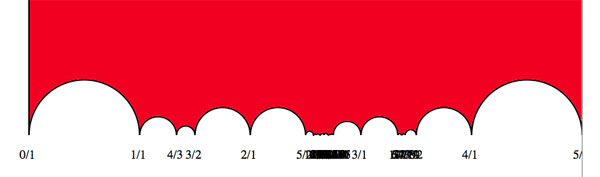the hiding place of $J_2$…

It would be nice to have (a) other Farey-symbols associated to the second Janko group, hopefully showing a pattern that one can extend into an infinite family as in the inguanodon series and (b) to determine Farey-symbols of more sporadic groups.

It’s been a while, so let’s include a recap : a (transitive) permutation representation of the modular group $\Gamma = PSL_2(\mathbb{Z})$ is determined by the conjugacy class of a cofinite subgroup $\Lambda \subset \Gamma$, or equivalently, to a dessin d’enfant. We have introduced a quiver (aka an oriented graph) which comes from a triangulation of the compactification of $\mathbb{H} / \Lambda$ where $\mathbb{H}$ is the hyperbolic upper half-plane. This quiver is independent of the chosen embedding of the dessin in the Dedeking tessellation. (For more on these terms and constructions, please consult the series Modular subgroups and Dessins d’enfants).

Why are quivers useful? To start, any quiver $Q$ defines a noncommutative algebra, the path algebra $\mathbb{C} Q$, which has as a $\mathbb{C}$-basis all oriented paths in the quiver and multiplication is induced by concatenation of paths (when possible, or zero otherwise). Usually, it is quite hard to make actual computations in noncommutative algebras, but in the case of path algebras you can just see what happens.

Moreover, we can also see the finite dimensional representations of this algebra $\mathbb{C} Q$. Up to isomorphism they are all of the following form : at each vertex $v_i$ of the quiver one places a finite dimensional vectorspace $\mathbb{C}^{d_i}$ and any arrow in the quiver
$$\xymatrix{\vtx{v_i} \ar[r]^a & \vtx{v_j}}$$ determines a linear map between these vertex spaces, that is, to $a$ corresponds a matrix in $M_{d_j \times d_i}(\mathbb{C})$. These matrices determine how the paths of length one act on the representation, longer paths act via multiplcation of matrices along the oriented path.

A necklace in the quiver is a closed oriented path in the quiver up to cyclic permutation of the arrows making up the cycle. That is, we are free to choose the start (and end) point of the cycle. For example, in the one-cycle quiver

$$\xymatrix{\vtx{} \ar[rr]^a & & \vtx{} \ar[ld]^b \\ & \vtx{} \ar[lu]^c &}$$

the basic necklace can be represented as $abc$ or $bca$ or $cab$. How does a necklace act on a representation? Well, the matrix-multiplication of the matrices corresponding to the arrows gives a square matrix in each of the vertices in the cycle. Though the dimensions of this matrix may vary from vertex to vertex, what does not change (and hence is a property of the necklace rather than of the particular choice of cycle) is the trace of this matrix. That is, necklaces give complex-valued functions on representations of $\mathbb{C} Q$ and by a result of Artin and Procesi there are enough of them to distinguish isoclasses of (semi)simple representations! That is, linear combinations a necklaces (aka super-potentials) can be viewed, after taking traces, as complex-valued functions on all representations (similar to character-functions).

In physics, one views these functions as potentials and it then interested in the points (representations) where this function is extremal (minimal) : the vacua. Clearly, this does not make much sense in the complex-case but is relevant when we look at the real-case (where we look at skew-Hermitian matrices rather than all matrices). A motivating example (the Yang-Mills potential) is given in Example 2.3.2 of Victor Ginzburg’s paper Calabi-Yau algebras.

Let $\Phi$ be a super-potential (again, a linear combination of necklaces) then our commutative intuition tells us that extrema correspond to zeroes of all partial differentials $\frac{\partial \Phi}{\partial a}$ where $a$ runs over all coordinates (in our case, the arrows of the quiver). One can make sense of differentials of necklaces (and super-potentials) as follows : the partial differential with respect to an arrow $a$ occurring in a term of $\Phi$ is defined to be the path in the quiver one obtains by removing all 1-occurrences of $a$ in the necklaces (defining $\Phi$) and rearranging terms to get a maximal broken necklace (using the cyclic property of necklaces). An example, for the cyclic quiver above let us take as super-potential $abcabc$ (2 cyclic turns), then for example

$\frac{\partial \Phi}{\partial b} = cabca+cabca = 2 cabca$

(the first term corresponds to the first occurrence of $b$, the second to the second). Okay, but then the vacua-representations will be the representations of the quotient-algebra (which I like to call the vacualgebra)

$\mathcal{U}(Q,\Phi) = \frac{\mathbb{C} Q}{(\partial \Phi/\partial a, \forall a)}$

which in ‘physical relevant settings’ (whatever that means…) turn out to be Calabi-Yau algebras.

But, let us return to the case of subgroups of the modular group and their quivers. Do we have a natural super-potential in this case? Well yes, the quiver encoded a triangulation of the compactification of $\mathbb{H}/\Lambda$ and if we choose an orientation it turns out that all ‘black’ triangles (with respect to the Dedekind tessellation) have their arrow-sides defining a necklace, whereas for the ‘white’ triangles the reverse orientation makes the arrow-sides into a necklace. Hence, it makes sense to look at the cubic superpotential $\Phi$ being the sum over all triangle-sides-necklaces with a +1-coefficient for the black triangles and a -1-coefficient for the white ones. Let’s consider an index three example from a previous post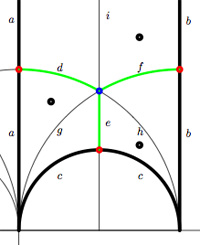$$\xymatrix{& & \rho \ar[lld]_d \ar[ld]^f \ar[rd]^e & \\ i \ar[rrd]_a & i+1 \ar[rd]^b & & \omega \ar[ld]^c \\ & & 0 \ar[uu]^h \ar@/^/[uu]^g \ar@/_/[uu]_i &}$$

In this case the super-potential coming from the triangulation is

$\Phi = -aid+agd-cge+che-bhf+bif$

and therefore we have a noncommutative algebra $\mathcal{U}(Q,\Phi)$ associated to this index 3 subgroup. Contrary to what I believed at the start of this series, the algebras one obtains in this way from dessins d’enfants are far from being Calabi-Yau (in whatever definition). For example, using a GAP-program written by Raf Bocklandt Ive checked that the growth rate of the above algebra is similar to that of $\mathbb{C}[x]$, so in this case $\mathcal{U}(Q,\Phi)$ can be viewed as a noncommutative curve (with singularities).

However, this is not the case for all such algebras. For example, the vacualgebra associated to the second index three subgroup (whose fundamental domain and quiver were depicted at the end of this post) has growth rate similar to that of $\mathbb{C} \langle x,y \rangle$…

I have an outlandish conjecture about the growth-behavior of all algebras $\mathcal{U}(Q,\Phi)$ coming from dessins d’enfants : the algebra sees what the monodromy representation of the dessin sees of the modular group (or of the third braid group).
I can make this more precise, but perhaps it is wiser to calculate one or two further examples…